Screenshots of the RAC Installation for ASM_HOME: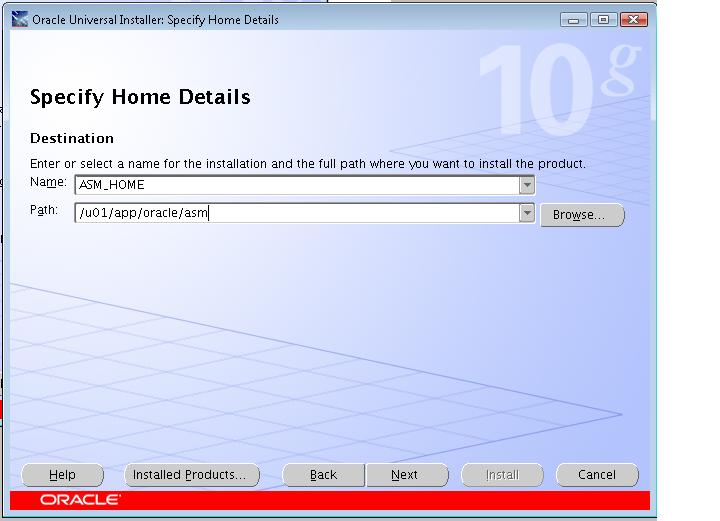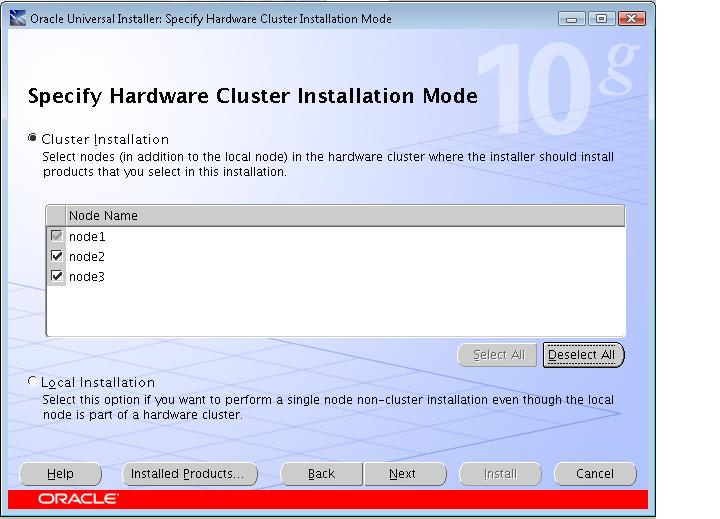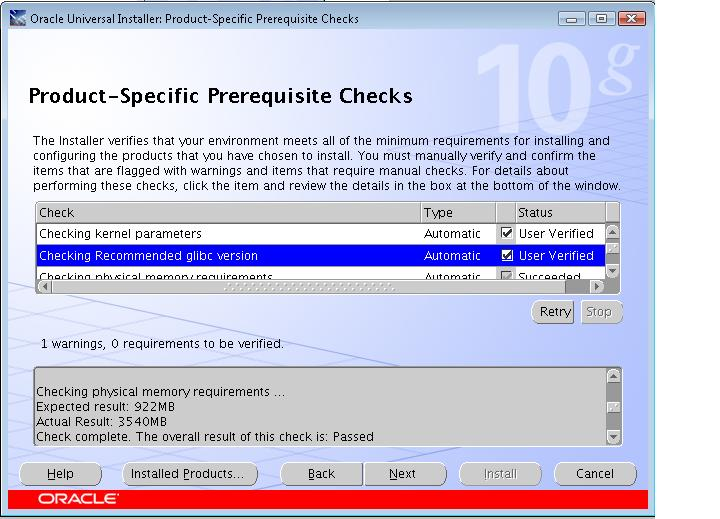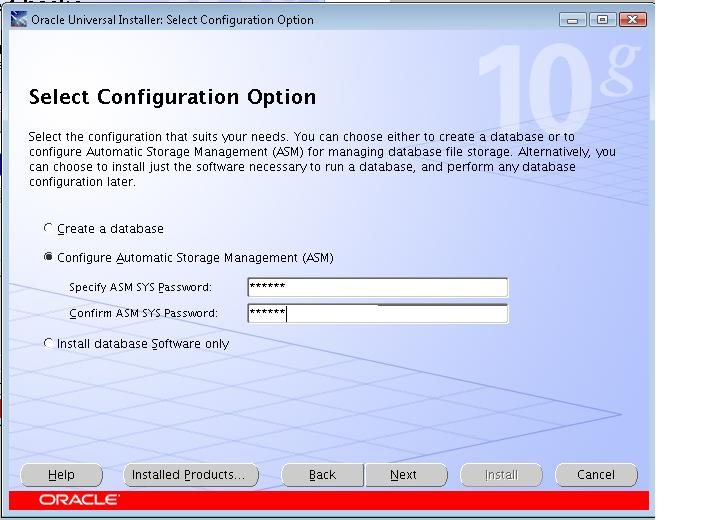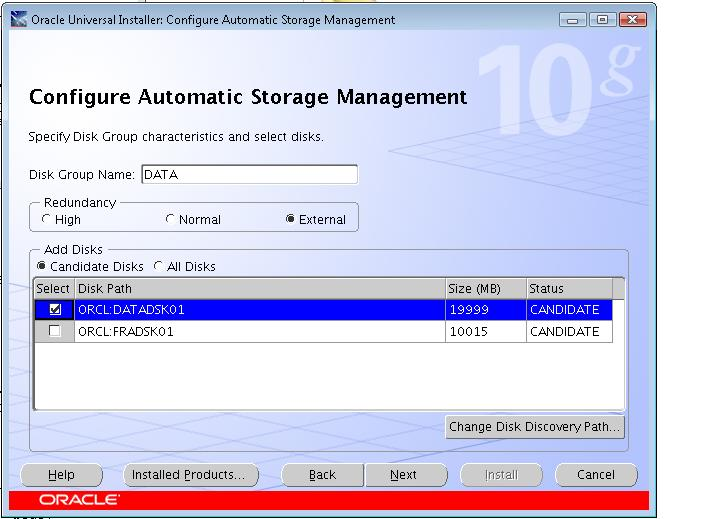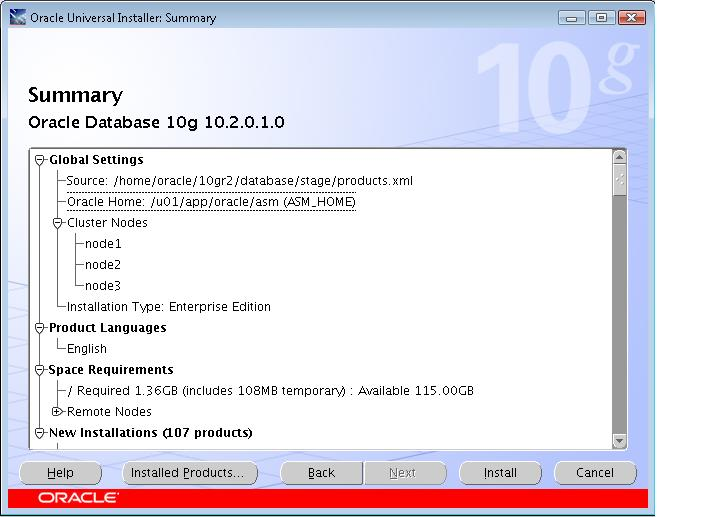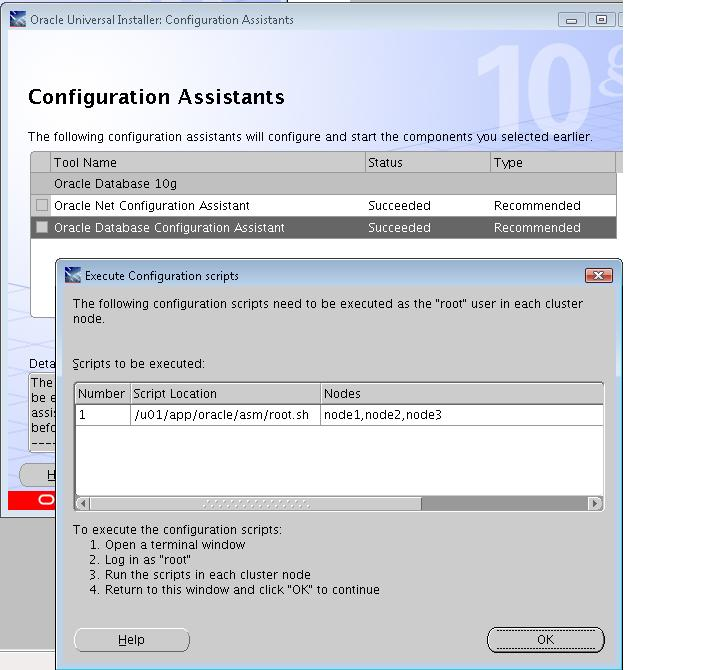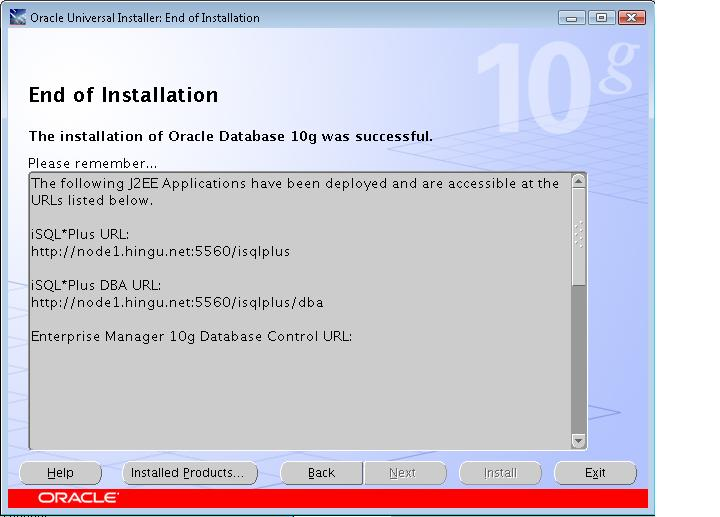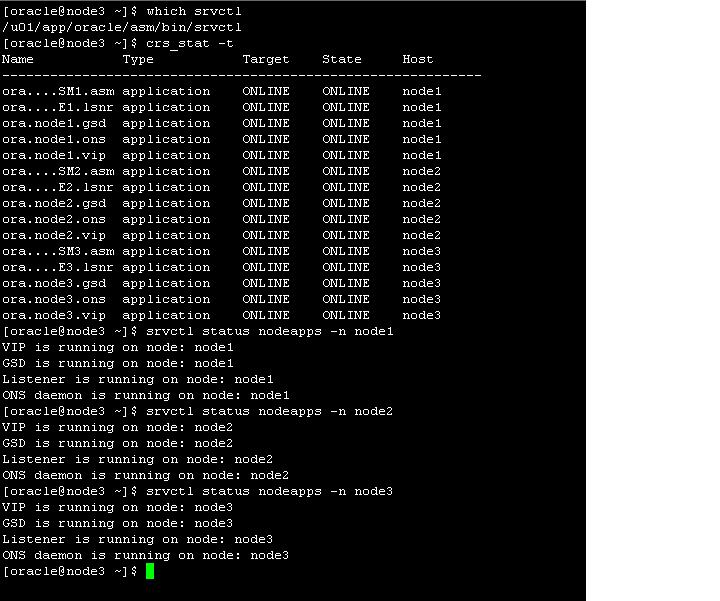Start the DBCA to create add the diskgroup FRA.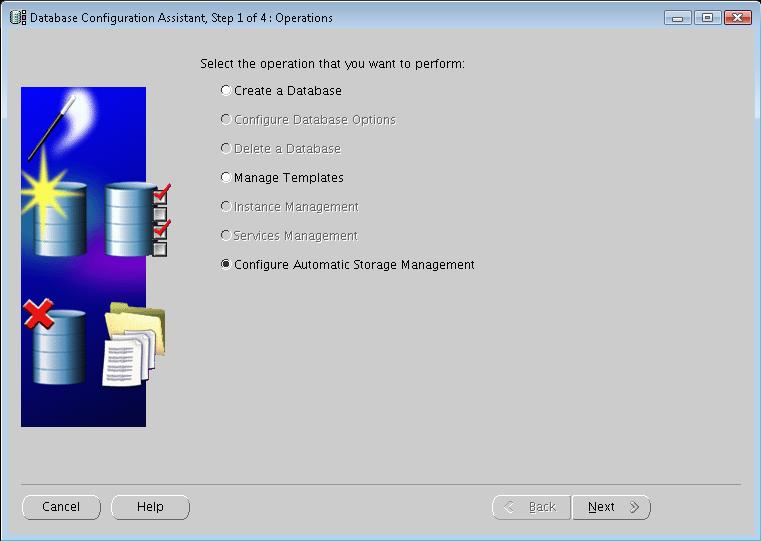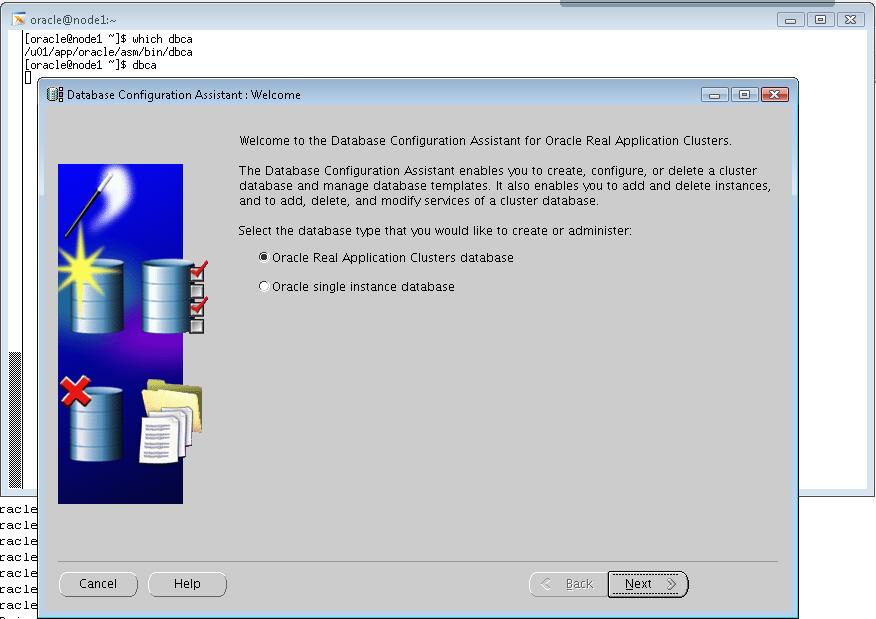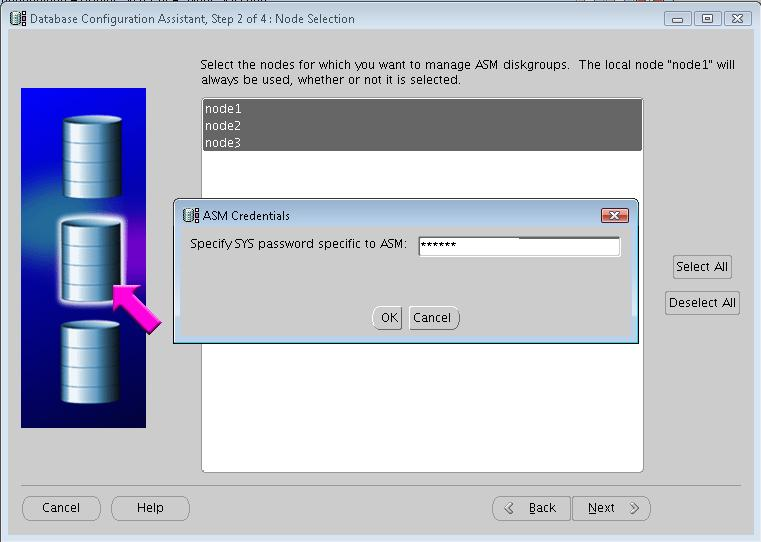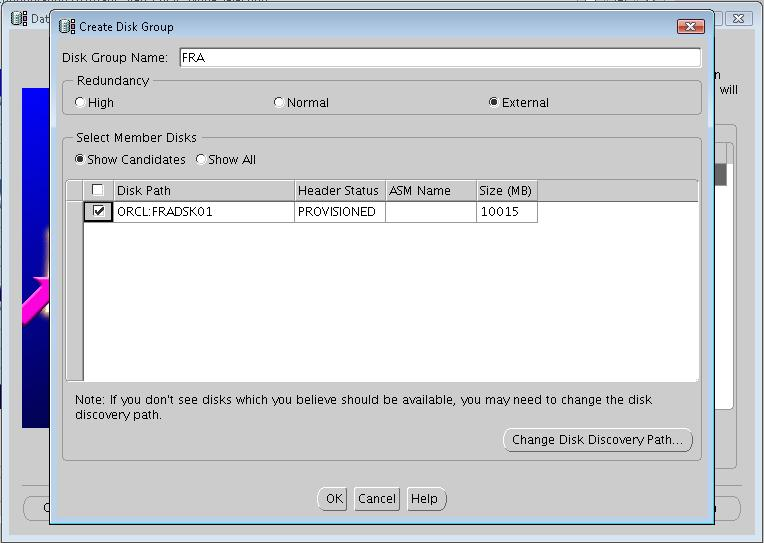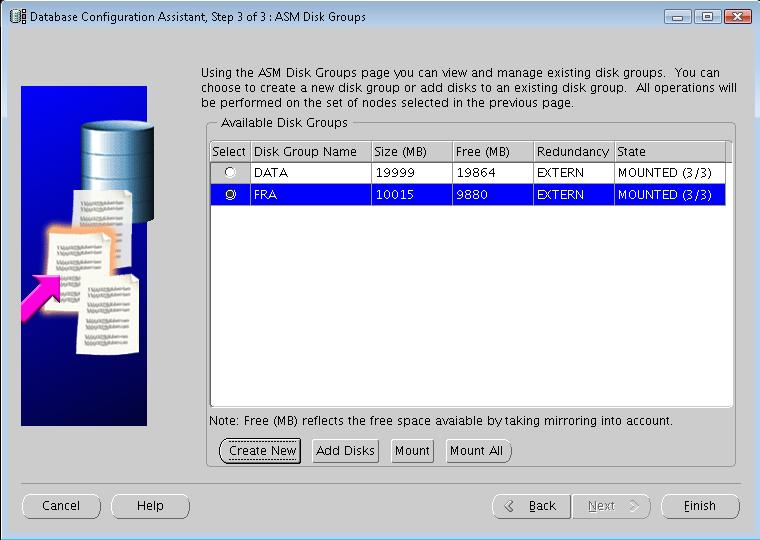tnsnames.ora (same on all the RAC nodes)

# tnsnames.ora.node2 Network Configuration File: /u01/app/oracle/asm/network/admin/tnsnames.ora.node2

# Generated by Oracle configuration tools.

EXTPROC_CONNECTION_DATA =

(DESCRIPTION =

(ADDRESS = (PROTOCOL = IPC)(KEY = EXTPROC1))

)

(CONNECT_DATA =

(SID = PLSExtProc)

(PRESENTATION = RO)

)

)

Listener.ora:

# listener.ora.node1 Network Configuration File: /u01/app/oracle/asm/network/admin/listener.ora.node1

# Generated by Oracle configuration tools.

SID_LIST_LISTENER_NODE1 =

(SID_LIST =

(SID_DESC =

(SID_NAME = PLSExtProc)

(ORACLE_HOME = /u01/app/oracle/asm)

(PROGRAM = extproc)

)

(SID_DESC =

(GLOBAL_DBNAME = +ASM)

(SID_NAME = +ASM1)

(ORACLE_HOME = /u01/app/oracle/asm)

)

)

LISTENER_NODE1 =

(DESCRIPTION_LIST =

(DESCRIPTION =

(ADDRESS = (PROTOCOL = TCP)(HOST = node1-vip.hingu.net)(PORT = 1521)(IP = FIRST))

(ADDRESS = (PROTOCOL = TCP)(HOST = 192.168.2.1)(PORT = 1521)(IP = FIRST))

)

)

# listener.ora.node2 Network Configuration File: /u01/app/oracle/asm/network/admin/listener.ora.node2

# Generated by Oracle configuration tools.

SID_LIST_LISTENER_NODE2 =

(SID_LIST =

(SID_DESC =

(SID_NAME = PLSExtProc)

(ORACLE_HOME = /u01/app/oracle/asm)

(PROGRAM = extproc)

)

(SID_DESC =

(GLOBAL_DBNAME = +ASM)

(SID_NAME = +ASM2)

(ORACLE_HOME = /u01/app/oracle/asm)

)

)

LISTENER_NODE2 =

(DESCRIPTION_LIST =

(DESCRIPTION =

(ADDRESS = (PROTOCOL = TCP)(HOST = node2-vip.hingu.net)(PORT = 1521)(IP = FIRST))

(ADDRESS = (PROTOCOL = TCP)(HOST = 192.168.2.2)(PORT = 1521)(IP = FIRST))

)

)

# listener.ora.node3 Network Configuration File: /u01/app/oracle/asm/network/admin/listener.ora.node3

# Generated by Oracle configuration tools.

SID_LIST_LISTENER_NODE3 =

(SID_LIST =

(SID_DESC =

(SID_NAME = PLSExtProc)

(ORACLE_HOME = /u01/app/oracle/asm)

(PROGRAM = extproc)

)

(SID_DESC =

(GLOBAL_DBNAME = +ASM)

(SID_NAME = +ASM3)

(ORACLE_HOME = /u01/app/oracle/asm)

)

)

LISTENER_NODE3 =

(DESCRIPTION_LIST =

(DESCRIPTION =

(ADDRESS = (PROTOCOL = TCP)(HOST = node3-vip.hingu.net)(PORT = 1521)(IP = FIRST))

(ADDRESS = (PROTOCOL = TCP)(HOST = 192.168.2.3)(PORT = 1521)(IP = FIRST))

)

)